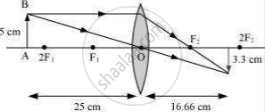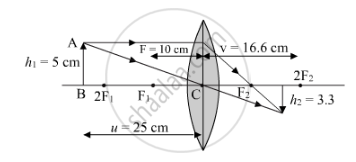# An object 5 cm in length is held 25 cm away from a converging lens of focal length 10 cm. Draw the ray diagram and find the position, size and the nature of the image formed. - Science

An object 5 cm in length is held 25 cm away from a converging lens of focal length 10 cm. Draw the ray diagram and find the position, size and the nature of the image formed.

#### Solution 1

Object distance, u = −25 cm
Object height, ho = 5 cm
Focal length, f = +10 cm
According to the lens formula,

1/v-1/u=1/f

1/v=1/f+1/u=1/10-1/25=15/250

v=250/15=16.66 cm

The positive value of v shows that the image is formed at the other side of the lens.

Magnification m =-"image distance"/"object distance"=-v/u=16.66/25=-0.66

The negative sign shows that the image is real and formed behind the lens.

Magnification m ="image height"/"object height"=H_i/H_o=H_i/5

Hi=m x Ho=-0.66 x 5=-3.3 cm

The negative value of image height indicates that the image formed is inverted.The position, size, and nature of image are shown in the following ray diagram.#### Solution 2

Given:
Object distance, u =-25 cm
Focal length, f = 10 cm
Height of the object, h = 5 cm
Applying the lens formula, we get:

1/f=1/v-1/u

⇒1/v=1/f+1/u

⇒1/v=1/f+1/u

⇒11/2/v=1/10+1/-25

⇒1/v=(5-2)/50

⇒1/v=3/50

⇒1/v=3/50

⇒v=50/3=+16.6  cm

The image will be at a distance of 16.6 cm behind the lens.

m=v/u=50/3

m=h_i/h=50/3

m=h_i/h=h_i/5

h_i/5=(-2)/3

h=(-10)/3=-3.3 cm

Thus, the image will be 3.3 high. It will be real and invertedConcept: Convex Lens
Is there an error in this question or solution?

#### APPEARS IN

NCERT Class 10 Science
Chapter 10 Light – Reflection and Refraction
Q 10 | Page 186
Lakhmir Singh Class 10 Physics (Science)
Chapter 5 Refraction of Light
Q 16 | Page 247